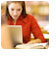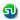### Linear equations, solutions, simultaneous equations, word problems. Algebra TANCET, XAT Quant sample Questions : Ascent TANCET, XAT Classes

 Home TANCET Classes GMAT Coaching Online GMAT Course CAT Classes TANCET Study Material
 TANCET '18 Classroom ProgramWeekend and weekday classes for TANCET MBA @ Chennai. At Nungambakkam and Velachery.Other Courses TANCET Correspondence Course CAT Classes & Courses GMAT Classes Chennai Online GMAT Course GRE Classes Chennai CBSE Math Online Tuition SAT Classes Other Links Ascent TANCET Toppers Testimonials CAT, TANCET Questions Careers @ Ascent Contact Us +91 44 4500 8484 +91 96000 48484 ascent@ascenteducation.com Postal Address Facebook / Twitter / Blog / Videos
You are here: Home  »  CAT, XAT, TANCET Prep Questions »  Linear Equations »   Question 4

# TANCET Quant Problems : (1st order) Linear Equations

The question for the day is a sample practice problem in Linear equation. Helps understand concepts related to formulating equations from a word problem and finding solution to the equations.

## Question

A railway half ticket costs half the full fare and the reservation charge is the same on half ticket as on full ticket. One reserved first class ticket from Chennai to Trivandrum costs Rs. 216 and one full and one half reserved first class tickets cost Rs. 327. What is the basic first class full fare and what is the reservation charge?
1. Rs. 105 and Rs. 6
2. Rs. 216 and Rs. 12
3. Rs. 210 and Rs. 12
4. Rs. 210 and Rs. 6

Choice (4). Rs. 210 and Rs. 6

Let half of the full basic fare be Rs. X.
Therefore, full basic fare is Rs. 2X.

Let the reservation charge be Rs. Y per ticket.
Now, one full reservation ticket would cost 2X (basic fare) + Y (reservation charge)
2X + Y = 216    --- (1)

The total basic fare for one half and one full ticket = X + 2X = 3X and the total reservation charge is 2Y.
Hence, 3X + 2Y = 327    --- (2)

Solving (1) and (2) we get,
X = 105 and Y = 6

Hence, the full basic fare is 2X = Rs. 210 and the reservation charge is Y = Rs. 6

## More Questions on Linear, Quadratic and Cubic Equations - Algebra

 5. 6. 7.

## CAT, XAT, TANCET Practice Questions and Answers : Listed Topicwise

 Number Theory Permutation Combination Probability Inequalities Geometry Mensuration Trigonometry Coordinate Geometry Percentages Profit Loss Ratio Proportion Mixtures Alligation Speed Time Distance Pipes Cisterns Interest Races Average, Mean AP, GP, HP Set Theory Clocks Calendars Algebra Function English Grammar General Awareness Data sufficiency TANCET Papers XAT PapersAdd to del.icio.usStumble Itdigg this